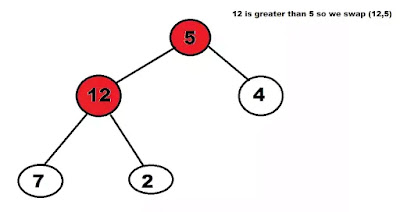heap is a specialized tree-based data structure and satisfies the below properties:

1. A Heap is a complete tree. what is a complete tree? in a complete tree all levels are completely filled except possibly the last level and the last level has all keys as left as possible.

2. A Heap is either Min Heap or Max Heap. In a Min-Heap, the key at root must be minimum among all keys present in the Binary Heap. The same property must be recursively true for all nodes in the Tree. In a Max-Heap, the key at root must be maximum among all keys present in the Binary Heap. The same property must be recursively true for all nodes in the Tree

Binary Heap: A Binary Heap is a heap where each node can have at most two children. a Binary Heap is nothing but a Binary Tree satisfying the above heap properties.

### min heap:

Binary Heaps representation:

they are represented using arrays:
• The root element will be at Arr.
• for  ith node, Arr[i]:
 Arr[(i-1)/2]  will be parent node Arr[(2*i)+1] will be the left child node Arr[(2*i)+2] will be the right child node

Finding Maximum Element: In a Max-Heap, the maximum element is always present at the root node and that is first element in the array which is used to represent the Heap. so maximum element Arr can be obtained in O(1) time complexity.

Finding Minimum ElementIn a Min-Heap, the minimum element is always present at the root node and that is first element in the array which is used to represent the Heap. so minimum element Arr can be obtained in O(1) time complexity.

Heapifying :

when we insert an element in heap, it doesnt follow the property of heap. so we place the element at the correct position to satisfy the Heap property this process is Heapify.

Heapifying in a Max Heap: In Max Heap every node's value must be greater than the values of its children nodes. So, to heapify a particular node swap the value of the node with the maximum value of its children nodes and continue the process until all of the nodes below it satisfies the Heap property.

consider the below heap as a Max-Heap:

In the above heap, node 5 does not follow the Heap property. Let's heapify the root node, node 5.
Step 1: Swap the node 5 with the maximum of its childrens i.e., max(12, 4).Step 2: Again the node 5 does not follow the heap property. Swap the node 5 with the maximum of its new childrens i.e., max(7, 2).

The Node 5 is now heapified successfully and placed at it's correct position.Time Complexity: The time complexity to heapify a single node is O(h), where h is equal to log(N) in a complete binary tree where N is the total number of nodes.

implementation (for max heap):
arr[] is an array
n is the size
i is the node to be heapified

in c++:
```void maxheapify(int arr[], int n, int i)
{
int largest = i; // Initialize largest as root
int l = 2 * i + 1; // left = 2*i + 1
int r = 2 * i + 2; // right = 2*i + 2

// If left child is larger than root
if (l < n && arr[l] > arr[largest])
largest = l;

// If right child is larger than largest so far
if (r < n && arr[r] > arr[largest])
largest = r;

// If largest is not root
if (largest != i) {
swap(arr[i], arr[largest]);

// Recursively heapify the affected sub-tree
maxheapify(arr, n, largest);
}
}```

in java:
```    static void maxheapify(int arr[], int n, int i)
{
int largest = i; // Initialize largest as root
int l = 2 * i + 1; // left = 2*i + 1
int r = 2 * i + 2; // right = 2*i + 2

// If left child is larger than root
if (l < n && arr[l] > arr[largest])
largest = l;

// If right child is larger than largest so far
if (r < n && arr[r] > arr[largest])
largest = r;

// If largest is not root
if (largest != i) {
int swap = arr[i];
arr[i] = arr[largest];
arr[largest] = swap;

// Recursively heapify the affected sub-tree
maxheapify(arr, n, largest);
}
}
```

similarly Heapifying can be done in a min Heap

implementation (for min heap):

in c++:
```void minheapify(int arr[], int n, int i)
{
int smallest = i; // Initialize smallest as root    int l = 2 * i + 1; // left = 2*i + 1
int r = 2 * i + 2; // right = 2*i + 2

// If left child is smaller than root
if (l < n && arr[l] < arr[smallest])
smallest = l;

// If right child is smaller than smallest so far    if (r < n && arr[r] < arr[smallest])
smallest = r;

// If smallest is not root    if (smallest != i) {
swap(arr[i], arr[smallest]);

// Recursively heapify the affected sub-tree
minheapify(arr, n, smallest);
}
}```

in java:
```    static void minheapify(int arr[], int n, int i)
{
int smallest = i; // Initialize smallest as root        int l = 2 * i + 1; // left = 2*i + 1
int r = 2 * i + 2; // right = 2*i + 2

// If left child is smaller than root        if (l < n && arr[l] < arr[smallest])            smallest = l;
// If right child is smaller than smallest so far        if (r < n && arr[r] < arr[smallest])            smallest= r;
// If smallest is not root        if (largest != i) {
int swap = arr[i];
arr[i] = arr[smallest];            arr[smallest] = swap;
// Recursively heapify the affected sub-tree
minheapify(arr, n, smallest);        }
}```

darkmode